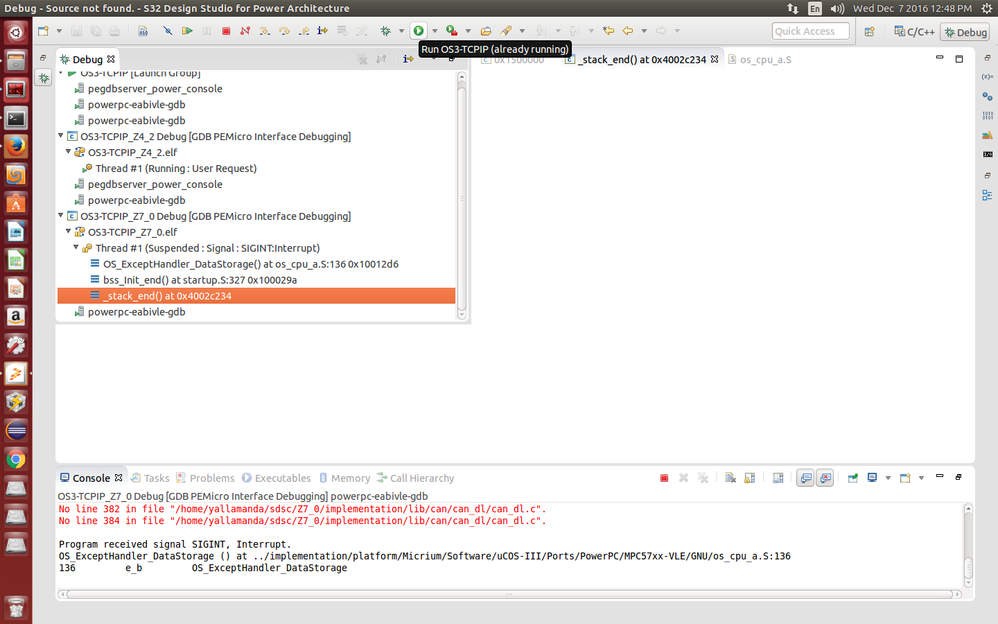# m_data is over flowed by 50k bytes MPC 5777M

cancel
Showing results for
Did you mean:

## m_data is over flowed by 50k bytes MPC 5777M

770 ViewsContributor V

Hi All, I am getting one error is m_data is over flowed by some 50k bytes, So as per one of the post in nxp community , I increased m_data section in 57xx_flash.ld file to 50k to the existing m_data which is (128k + 50k). So I am able to compile it , but when i flashed the code on to mpc5777m board , it is not at all working  and I am able to see the error _stack_end at/* Entry Point */
ENTRY(_start)

/* define heap and stack size */
__HEAP_SIZE            = 0 ;
__STACK_SIZE           = 4096 ;

/* Define Flash Base Address */
FLASH_SIZE =  2560K;

/* Define SRAM Base Address */
/*SRAM_SIZE =  128K;*/
SRAM_SIZE =  178K;    (added 50k here)

/* Define Caching Inhibited RAM Base Address */
MPU_NOCACHE_SIZE = 8K;
/* Define CPU0 Local Instruction SRAM Base Address */
LOCALIMEM_SIZE = 16K;

/* Define CPU0 Local Data SRAM Base Address */
LOCALDMEM_SIZE = 64K;

MEMORY
{

flash_rchw : org = 0x00FC0000,   len = 0x4
cpu0_reset_vec : org = 0x00FC0000+0x10,   len = 0x4
cpu1_reset_vec : org = 0x00FC0000+0x14,   len = 0x4
cpu2_reset_vec : org = 0x00FC0000+0x04,   len = 0x4
cpuc_reset_vec : org = 0x00FC0000+0x18,   len = 0x4

m_text :    org = 0x1000000, len = 2560K
m_data :    org = 0x40000000,   len = 178K
m_data_nocache :    org = 0x4017B4E0,   len = 8K

int_iram  : org = 0x50000000,   len = 16K
int_dram  : org = 0x50800000,   len = 64K
}

I am not getting , how to solve this problem , can any one help me ?

Labels (1)
• ### General

Tags (4)
7 Replies
426 ViewsNXP Employee

Hi,

Have you created single-core or multi-core project? If you create multi-core project, could you please send me linker files for all cores?

Regards,

Martin

426 ViewsContributor V

Hi I have two cores one is z7_0 and z4_2 . the one is which is posted in first query is for Z7_0 and for the z4_2 is given below :

/* Entry Point */
ENTRY(_start)

/* define heap and stack size */
__HEAP_SIZE            = 0 ;
__STACK_SIZE           = 4096 ;

SRAM_SIZE =  128K;
/* Define SRAM Base Address */

/* Define CPU2 Local Instruction SRAM Allocation */
LOCALIMEM_SIZE = 16K;
/* Define CPU2 Local Instruction SRAM Base Address */

/* Define CPU2 Local Data SRAM Allocation */
LOCALDMEM_SIZE = 64K;
/* Define CPU2 Local Data SRAM Base Address */

MEMORY
{

flash_rchw : org = 0x00FC0000,   len = 0x4
cpu0_reset_vec : org = 0x00FC0000+0x10,   len = 0x4
cpu1_reset_vec : org = 0x00FC0000+0x14,   len = 0x4
cpu2_reset_vec : org = 0x00FC0000+0x04,   len = 0x4
cpuc_reset_vec : org = 0x00FC0000+0x18,   len = 0x4

m_text :    org = 0x1500000, len = 2560K
m_data :    org = 0x40040000,   len = 128K

int_iram  : org = 0x52000000,   len = 16K
int_dram  : org = 0x52800000,   len = 64K
}

426 ViewsNXP Employee

Hi,

just a quick hint. This line in linker file seems to be incorrect. MPU_NOCACHE_BASE_ADDR = 0x4017B4E0; You started your SRAM at address 0x40000000 and length is 178KB, so address range is 0x40000000 - 0x4002C800.

From the picture you shared it seems, you got Data Storage exception and the incorrect address could be the root cause of this exception.

Regards,

Martin

426 ViewsContributor V

Hi Martin, I am able to run the code on the board only after increasing SRAM size to 256k, but only two tasks are  running , we have three tasks in my code (micrium rtos tasks). So I disable one task. Can I increase to some more ( to 300k) but MPC5777M data sheet tells onchip SRAM is 404KB , I have two cores running ,one (Z4_2) I configured 128KB SRAM and   another one Z7_0 for 256KB.

To what extent I can increase SRAM size or any other option to get rid off this ?b55689

426 ViewsNXP Employee

Hi,

unfortunately, I do not know Micrium RTOS, but you have only one possible way. If you do not have enough memory for operating system, you can decrease the SRAM size for Z4 core (if you do not need all 128K for Z4 core).

Otherwise you have to optimize your core.

Regards,
Martin

426 ViewsContributor V

Hi Martin Thank you ,I will check it.b55689

426 ViewsContributor V

Thank you I got it ,Actually I am multiplying (178KB) 178*1024 in hexadecimal .

I will check the same.